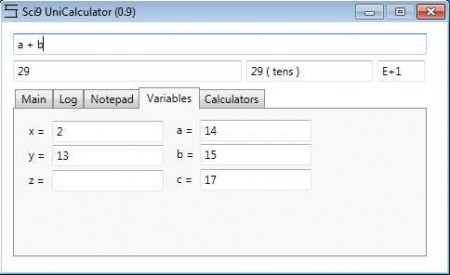Editor Ratings:
User Ratings:
[Total: 0 Average: 0]

Sci9 Calculator is a very advanced calculator application for the Windows desktop environment which offers pretty much everything from simple additions to the more advanced matrices and vectors. Students are always in need of a decent calculator application, which has advanced scientific mathematical functions, now with Sci9 Calculator you can get all of that rolled up in a very small app.As you can see from the image above, the Sci9 Calculator interface is very advanced, additional options are hidden with the help of 5 tabs, and at the top we also have additional text boxes, beneath the main one, where Sci9 is gonna display automatically the results of the calculations, but also offer you some useful things like textual representations of results and shorter record of the results with the help of exponents. Key features of Sci9 Calculator are:

• 4 different modes of work – Radians, Degrees, Grads, Full Circle
• 21 different functions – roots, exponents, trigonometry, algorithms etc
• Matrix, vector 2D and 3D, roots, base converter calculators
• Calculate equations with up to 6 different variables
• Constants – Pi, Golden ration, Euler’s number, Plastic constant etc.

A very useful feature that Sci9 Calculator has would be the Notepad, where you can add various different doodles and in between work, the leg work before deciding to commit to anything and before actually starting to type in the mathematical functions in the main window at the top of the application. Here’s a few tips on how you can do that.

Similar software: Free Calculator, Tibi’s Mathematical Suite, SpeQ Mathematics

## How to perform calculations with Sci9 Calculator

After download, simply run Sci9 Calculator and it should start working right away. Longest input bar at the top is where you can enter the mathematical functions that interest you.Let’s say that you would like to deal with variables, to do so open up the variables tab, once you’re there, enter the values that your variables have and then simply start using them in your calculations at the top. Every time that you write the variable, it’s gonna assume the number which you gave to it. Keep the same calculation, just exchange the numbers of the variable if you want to calculate different numbers.To get all the roots that you can get from a certain number, you’re gonna have to select the Get Roots option in the Calculators tab. In this section you can find a lot of different useful calculators like the one for matrices, vectors and among other things there’s also a tool which you can use in order to check if the number which you’ve entered or calculated is really a Prime number.

## Conclusion

Even though the calculator which can be found in Windows is very advanced, it’s not as advanced as Sci9 Calculator, which I’m sure you’ll agree with after you spend some time, playing around with this advanced scientific calculator. The best thing about Sci9 is that there’s also an online version of it, which you can access only on the website. Download this free scientific calculator, if you go to school or college, it can be very useful.